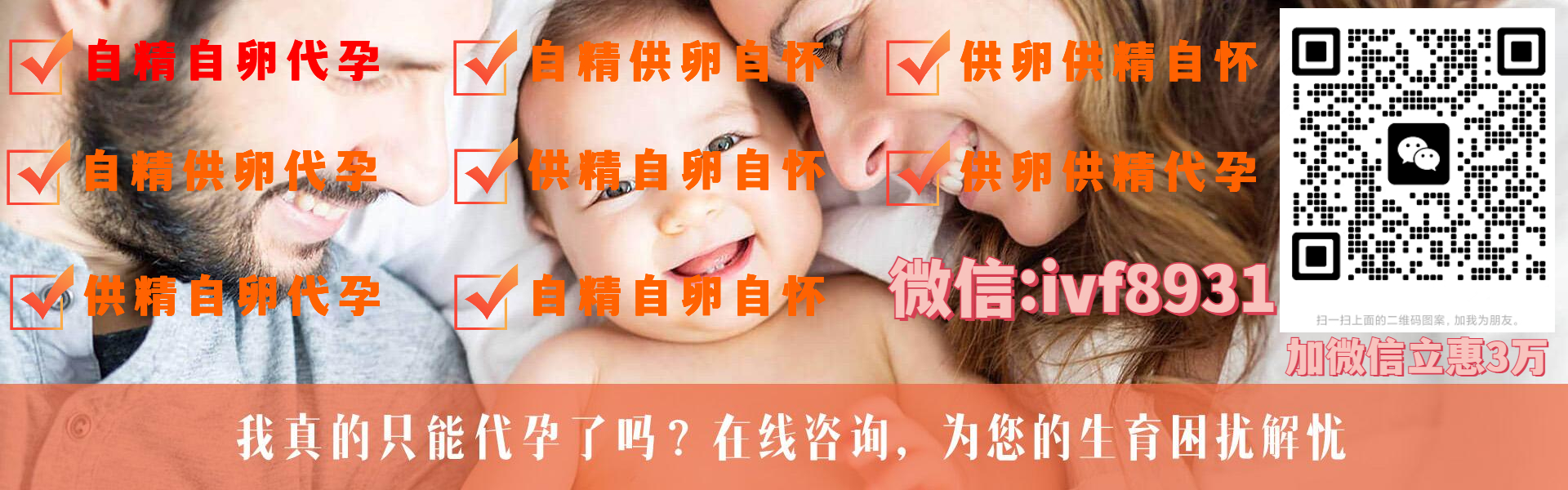# 用Python爬了微信好友，原來他們是這樣的人

2021年12月10日15:16:05 1714

• itchat： 微信網頁版介面封裝Python版本，在本文中用以獲取微信好友信息。
• jieba： 結巴分詞的 Python 版本，在本文中用以對文本信息進行分詞處理。
• matplotlib： Python 中圖表繪製模塊，在本文中用以繪製柱形圖和餅圖
• snownlp： 一個 Python 中的中文分詞模塊，在本文中用以對文本信息進行情感判斷。
• PIL： Python 中的圖像處理模塊，在本文中用以對圖片進行處理。
• numpy： Python中 的數值計算模塊，在本文中配合 wordcloud 模塊使用。
• wordcloud： Python 中的詞雲模塊，在本文中用以繪製詞雲圖片。
• TencentYoutuyun： 騰訊優圖提供的 Python 版本 SDK ，在本文中用以識別人臉及提取圖片標籤信息。

01

## 數據分析

friends = itchat.get_friends(update = True)## 好友性別

def analyseSex(firends):

sexs = list(map(lambda x:x['Sex'],friends[1:]))

counts = list(map(lambda x:x,Counter(sexs).items()))

labels = ['Unknow','Male','Female']

colors = ['red','yellowgreen','lightskyblue']

plt.figure(figsize=(8,5), dpi=80)

plt.axes(aspect=1)

plt.pie(counts, #性別統計結果

labels=labels, #性別展示標籤

colors=colors, #餅圖區域配色

labeldistance = 1.1, #標籤距離圓點距離

autopct = '%3.1f%%', #餅圖區域文本格式

startangle = 90, #餅圖起始角度

pctdistance = 0.6 #餅圖區域文本距離圓點距離

)

plt.legend(loc='upper right',)

plt.title(u'%s的微信好友性別組成' % friends['NickName'])

plt.show()03

## 好友頭像

# Init Path

basePath = os.path.abspath('.')

if(os.path.exists(baseFolder) == False):

os.makedirs(baseFolder)

# Analyse Images

faceApi = FaceAPI()

use_face = 0

not_use_face = 0

image_tags = ''

for index in range(1,len(friends)):

friend = friends[index]

imgfile = baseFolder + '\\Image%s.jpg' % str(index)

if(os.path.exists(imgFile) == False):

with open(imgFile,'wb') as file:

file.write(imgData)

# Detect Faces

time.sleep(1)

result = faceApi.detectFace(imgFile)

if result == True:

use_face += 1

else:

not_use_face += 1

# Extract Tags

result = faceApi.extractTags(imgFile)

image_tags += ','.join(list(map(lambda x:x['tag_name'],result)))

labels = [u'使用人臉頭像',u'不使用人臉頭像']

counts = [use_face,not_use_face]

colors = ['red','yellowgreen','lightskyblue']

plt.figure(figsize=(8,5), dpi=80)

plt.axes(aspect=1)

plt.pie(counts, #性別統計結果

labels=labels, #性別展示標籤

colors=colors, #餅圖區域配色

labeldistance = 1.1, #標籤距離圓點距離

autopct = '%3.1f%%', #餅圖區域文本格式

startangle = 90, #餅圖起始角度

pctdistance = 0.6 #餅圖區域文本距離圓點距離

)

plt.legend(loc='upper right',)

plt.title(u'%s的微信好友使用人臉頭像情況' % friends['NickName'])

plt.show()

image_tags = image_tags.encode('iso8859-1').decode('utf-8')

back_coloring = np.array(Image.open('face.jpg'))

wordcloud = WordCloud(

font_path='simfang.ttf',

background_color="white",

max_words=1200,

max_font_size=75,

random_state=45,

width=800,

height=480,

margin=15

)

wordcloud.generate(image_tags)

plt.imshow(wordcloud)

plt.axis("off")

plt.show()## 好友簽名

def analyseSignature(friends):

signatures = ''

emotions = []

pattern = re.compile("1f\d.+")

for friend in friends:

signature = friend['Signature']

if(signature != None):

signature = signature.strip().replace('span', '').replace('class', '').replace('emoji', '')

signature = re.sub(r'1f(\d.+)','',signature)

if(len(signature)>0):

nlp = SnowNLP(signature)

emotions.append(nlp.sentiments)

signatures += ' '.join(jieba.analyse.extract_tags(signature,5))

with open('signatures.txt','wt',encoding='utf-8') as file:

file.write(signatures)

# Sinature WordCloud

back_coloring = np.array(Image.open('flower.jpg'))

wordcloud = WordCloud(

font_path='simfang.ttf',

background_color="white",

max_words=1200,

max_font_size=75,

random_state=45,

width=960,

height=720,

margin=15

)

wordcloud.generate(signatures)

plt.imshow(wordcloud)

plt.axis("off")

plt.show()

wordcloud.to_file('signatures.jpg')

# Signature Emotional Judgment

count_good = len(list(filter(lambda x:x>0.66,emotions)))

count_normal = len(list(filter(lambda x:x>=0.33 and x<=0.66,emotions)))

labels = [u'負面消極',u'中性',u'正面積極']

plt.rcParams['font.sans-serif'] = ['simHei']

plt.rcParams['axes.unicode_minus'] = False

plt.xlabel(u'情感判斷')

plt.ylabel(u'頻數')

plt.xticks(range(3),labels)

plt.legend(loc='upper right',)

plt.bar(range(3), values, color = 'rgb')

plt.title(u'%s的微信好友簽名信息情感分析' % friends['NickName'])

plt.show()## 05

def analyseLocation(friends):

with open('location.csv','w',encoding='utf-8',newline='',) as csvFile:

for friend in friends[1:]:

row = {}

row['NickName'] = friend['NickName']

row['Province'] = friend['Province']

row['City'] = friend['City']

writer.writerow(row)06

## 總結## 華為秋季發布會不提mate60和麒麟晶元？是沒做好全面反擊？

9月25日，華為召開了隆重的全場景新品發布會，全程140多家媒體跟蹤直播。不過發布會剛結束，就有人說全程不提mate60和麒麟晶元的事，讓人有點失望，這是這樣么？

## Mixin疑被盜，虛擬幣大跌！幣圈再次慌了

#時事熱點頭條說#有投資者表示，今天突然收到通知，Exin 團隊關於 Mixin Network 雲服務商資料庫遭受攻擊的處理辦法。

## 華為WATCH GT 4正式發布，全新高端腕錶設計引領腕間時尚

9月25日，華為秋季全場景新品發布會如約而至，華為WATCH GT 4系列正式發布，在標誌性長續航的基礎上全面進化。華為WATCH GT 4系列擁有46mm和41mm兩種規格，分別採用八邊稜角設計和閃耀光環設計，使手錶在圓形錶盤的基礎上更具細節和質感；搭載全新TruSeen™5.